Courses

# Magnetostatics (Part - 4) Electrical Engineering (EE) Notes | EduRev

## Electrical Engineering (EE) : Magnetostatics (Part - 4) Electrical Engineering (EE) Notes | EduRev

The document Magnetostatics (Part - 4) Electrical Engineering (EE) Notes | EduRev is a part of the Electrical Engineering (EE) Course Electromagnetic Theory.
All you need of Electrical Engineering (EE) at this link: Electrical Engineering (EE)

Boundary Conditions at Interface

We have seen that the normal component of the electric field (and hence the electric field itself) is discontinuous a charged surface. In a similar way the magnetic field is coscontinuous across a surface which has surface current.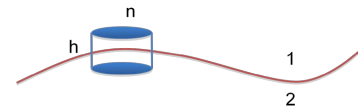Consider interface of two regions, marked 1 and 2. A surface current flows which comes out of the plane of the paper.

Consider a thin pillbox of height h and area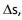perpendicular to the media with half below the surface and half above it. According to magnetostatic Gauss’s theorem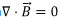can be expressed as a surface integral,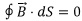Define the normal direction as the outward direction from the surface into the region 1. As h→0, contributions to the surface integral only comes from the top and the bottom caps, so that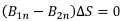which shows that the normal component of magnetic induction is continuous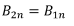In an identical fashion, it follows that since in Coulomb gauge, we have,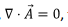the normal component of the vector potential is continuous,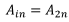The tangential component, i.e. component of the magnetic field parallel to the interface has a discontinuity which can be calculated by taking a rectangular Amperian loop of length L and a negligible height h with its length being parallel to the interface. The current density, as in the first case comes out of the plane of the paper.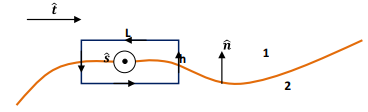Let us define our coordinate system . In the figure shown, the interface is perpendicular to the page and the normal to the surface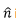is outward into the region 1. The unit vectors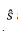and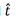Are both in the plane of the interface, withcoming out of the page and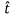also on the surface perpendicular toso thatandform a right handed triad.

Let us calculate the line integral around the loop. Taking the long side of the loop to be parallel to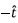direction, the line integral of the magnetic field is (as h→0)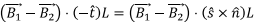By Ampere’s law, this should be equal to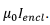Since the current flows on the surface and the normal to the loop is alongwe have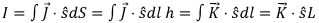where we have used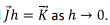Equating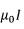to the line integral obtained earlier, we have,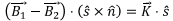Using the cyclic property of scalar triple product for the expression to the left and reversing the order of the dot product on the right , we can rewrite the left hand side and get,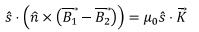which gives,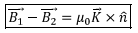[This can be seen by substituting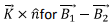in the preceding expression and realizing that since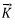lies on the interface,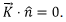This expression also includes the boundary condition on the normal component as well because the normal component of the right hand side is identically zero.

What about the boundary condition on the vector potential? We have seen that the normal component of the vector potential is continuous because of our choice of gauge. The tangential component is also continuous because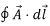being equal to the magnetic flux through the plane of the loop is zero. Thus both the tangential and the normal component of the vector potential are continuous.

The discontinuity in the tangential component of the magnetic field, however, translates to a discontinuity in the normal derivative of the vector potential. Proof of this is left as an exercise.

Magnetic Scalar Potential

We had seen that since the curl of the magnetic field is non-zero, the field is non-conservative as a result of which, unlike in the case of the electrostatic field, we cannot define a scalar potential. However, if we restrict ourselves to region other than where a current source exists, the curl would be zero. In such a situation, we can define a scalar potential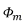corresponding to the magnetic field in the region.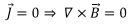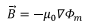Along with the divergence for the magnetic field being zero, this leads to Laplace’s equation for the scalar magnetic potential,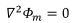The equation is similar to the case of electrostatic potential. The multiplicative factor μhas been introduced because of dimensional reasons and will be clarified later.

Example : Scalar potential for a line current

The problem has cylindrical symmetry. Taking the direction of the current along the z direction, the magnetic field is given by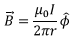where r is the distance of the point of observation from the wire. Expressing the equation defining the scalar potential in cylindrical coordinates, we have,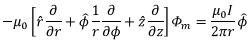Only the second term of the left hand expression can give us the desired magnetic field,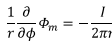This gives,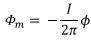The scalar potential is thus proportional to the polar angle. The potential is multiple valued because as the origin is circled more than once, the angle increases by 2π.

Example : Scalar Potential for a Magnetic Dipole :

In the previous lecture, we have obtained expressions for the components of a magnetic dipole directed along the z direction. We have seen that the magnetic field can be written as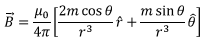Writing the gradient in spherical polar and equating the components, we have,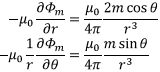Both these equations are satisfied if we choose,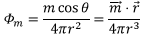Consider the magnetic moment to be generated by a circular current loop carrying a current I. Taking the direction of the magnetic moment along the z direction, he point of observation makes an angle θ with the normal to the plane of the loop. We can express this in terms of the solid angle subtended by the current loop.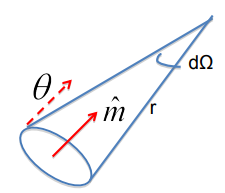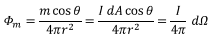The solid angle can be both positive and negative depending on the way the loop is viewed from the point of observation.

A comment about singlevaluedness of the scalar potential is appropriate here. When we take the line integral of the magnetic field, there is no discontinuity if the loop does not enclose any current. Thus as the point of observation is changed, there will be a discontinuity if it posses through the loop. In the following, the current loop is made to be the rim of an open surface, the shape of the surface is immaterial as long as the rim is the current loop. If we take any loop on this surface , there is no discontinuity if the loop does not go around the rim. If the shape is taken to be a hemisphere, the loop cannot start from a point on the upper hemisphere and pass on to the lower hemisphere. If the loop does pass through the current, we have,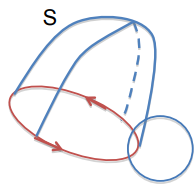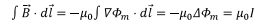so that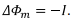This is consistent with the fact that every time the loop is enclosed, the solid angle changes by 4π.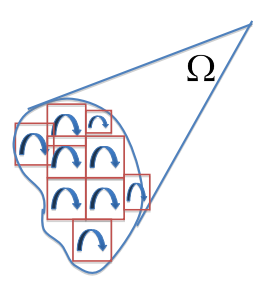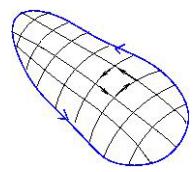What about an arbitrary loop? We need to find the solid angle it makes at the point of observation. We can divide the current loop into a large mesh of small loops, each one of which behaves like a magnetic moment. The directions of adjacent loops cancel leaving only the contour of the loop, as shown. Since the discontinuity in the scalar potential when we traverse a current loop once is –I, If we traverse the loop so that it traces a solid angle Ω at the point of observation, the scalar potential would change by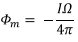Tutorial Assignment

1. Prove that the normal derivative of the vector potential is discontinuous across a surface carrying current.
2. Calculate the scalar potential due to a solenoid on its axis at a distance z from the centre of the solenoid.

Solutions to Tutorial Assignments

1. We have shown that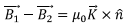In terms of vector potential, this can be written as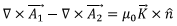Take the cross product of both sides with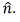To do so note that we can simplify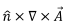as follows. Expanding in terms of Cartesian components,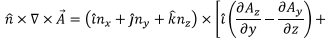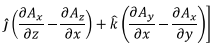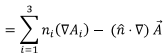where,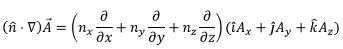Thus,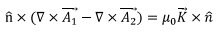gives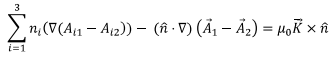We hade seen that each component of the vector potential is continuous at the boundary. Thus the first term must be zero. The tangential component of the second term is obviously zero which leaves us with only the normal component in the above equation. Once again the first term is zero, giving,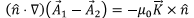2. Choose the origin at the centre of the solenoid of length L. In Problem 1 of the Self Assessment Quiz, you will prove that the scalar potential at a distance z is given by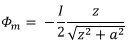Let the number of turns per unit length be n. Consider a width dz’ of the solenoid located at z’ from the centre. The potential due to this is given by as the centre of the loop is shifted to z’)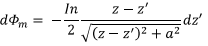The total potential at z is obtained by integrating this expression from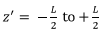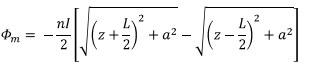Self Assessment Questions

1. Calculate the magnetic scalar potential at a distance z from a circular current loop of radius a and hence calculate the magnetic field at that point.
2. A current sheet of 10î (in kA/m) separates two regions of space at z=0. The magnetic field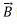in region 1 (z>0) is given by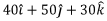(in mT) exists. If both regions are non-magnetic, determine the field in region 2 (z<0).

Solutions to Self Assessment Questions

1. The problem is essentially to calculate the solid angle subtended by the current loop at a distance z from its centre. With the point where we need the scalar potential as the     centre draw a sphere of radius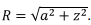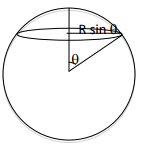It is easy to show that the part of the sphere above the loop has an area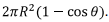Since an area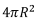subtends a solid angle 4π , the loop subtends 2π(1-Cosθ).In terms of the distance z from the centre of the loop, the solid angle is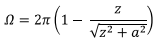Thus the scalar potential is given by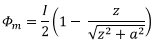Thus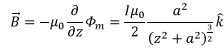2. This is a simple application of the magnetostatic boundary condition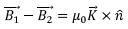In this case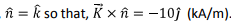Multiplying with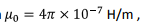we get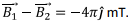Thus the magnetic field of induction in the second medium is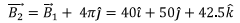Offer running on EduRev: Apply code STAYHOME200 to get INR 200 off on our premium plan EduRev Infinity!

,

,

,

,

,

,

,

,

,

,

,

,

,

,

,

,

,

,

,

,

,

;